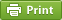﻿ How to draw Lewis structures

# How to draw Lewis structures## Step method to draw lewis structures

Drawing of lewis structures involves the calculation of valence electrons and putting them in a dotted structure.These structures are used to represent the valence electrons involved in forming a chemical compound.
Rule 1: Every atom should get 8 electrons in any chemical compound. This is known as octet rule.
The following elements do not obey the octet rule and their octet values are as follows:
H=2
He=2
Li=4
Be=4
B=6
Some compounds form double and triple bonds. An extra pair of electrons can be shared between the central atom and side atom to form double bond, and 2 pairs to form a triple bond.
Step method to draw lewis structures. ( ammonia(NH3) is used as an example)
Step 1: Find valence e- in all atoms. Add them together.

N=5
H=1x3=3
Total=8

Step2: Find octet e- for each atom and add them together.
N=8
H=2x3=6
Total=14

Step3: Subtract step 1 total from step 2.This step gives you bonding e-.
14-8=6e-

Step 4: Divide the number in step 3 by 2(because each bond is made of 2 e-).This step gives you number of bonds.

6e-/2= 3 bond pairs

Step 5: Subtract step 3 number from step 1.This step gives you number of nonbonding (lone pairs) e-.
8-6= 2e-=1 lone pair

Use information from step 4 and 5 to draw the lewis structure.
Put atom with lowest electro negativity in the center. Arrange the remaining atoms around it. Finally put the bond pairs and lone pairs of electrons on the atoms.Nitrogen goes in the centre.## Lewis dot structure

Alternatively a dot method can be used to draw the lewis structure.The following method shows you how to draw dot structure for ammonia.
Calculate the total valence electrons in NH3 molecule.
N=5,
H=1x3=3
Total=8

Put Nitrogen in the center and three hydrogen atoms on the sides.
Put a pair of electrons connecting the side atom with central atom.Put the fourth pair on the central atom,Nitrogen.